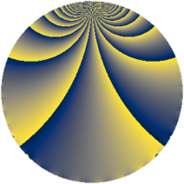# Properties

 Label 1520.2.bnLevel $1520$ Weight $2$ Character orbit 1520.bn Rep. character $\chi_{1520}(37,\cdot)$ Character field $\Q(\zeta_{4})$ Dimension $472$ Sturm bound $480$

# Related objects

## Defining parameters

 Level: $$N$$ $$=$$ $$1520 = 2^{4} \cdot 5 \cdot 19$$ Weight: $$k$$ $$=$$ $$2$$ Character orbit: $$[\chi]$$ $$=$$ 1520.bn (of order $$4$$ and degree $$2$$) Character conductor: $$\operatorname{cond}(\chi)$$ $$=$$ $$1520$$ Character field: $$\Q(i)$$ Sturm bound: $$480$$

## Dimensions

The following table gives the dimensions of various subspaces of $$M_{2}(1520, [\chi])$$.

Total New Old
Modular forms 488 488 0
Cusp forms 472 472 0
Eisenstein series 16 16 0

## Trace form

 $$472q + 8q^{4} - 4q^{5} - 8q^{6} + 456q^{9} + O(q^{10})$$ $$472q + 8q^{4} - 4q^{5} - 8q^{6} + 456q^{9} - 8q^{11} - 16q^{16} - 8q^{17} + 8q^{19} + 8q^{20} - 24q^{24} - 24q^{26} - 32q^{28} - 16q^{30} - 8q^{35} - 8q^{36} - 32q^{38} - 48q^{39} - 8q^{43} + 16q^{44} - 36q^{45} - 8q^{47} - 56q^{54} - 12q^{57} + 24q^{58} - 40q^{61} + 24q^{62} - 64q^{63} + 32q^{64} + 56q^{66} + 40q^{68} - 48q^{74} - 36q^{76} + 48q^{77} - 16q^{80} + 392q^{81} + 88q^{82} + 16q^{85} - 24q^{87} - 80q^{92} + 20q^{95} + 80q^{96} + 8q^{99} + O(q^{100})$$

## Decomposition of $$S_{2}^{\mathrm{new}}(1520, [\chi])$$ into newform subspaces

The newforms in this space have not yet been added to the LMFDB.